Publication date: 04/12/2021

## Run the Design and Analyze the Results

In this section, you analyze the results obtained when you obtain results from the final design. In particular, you want to know how changing the price or other characteristics of a laptop affects its desirability as perceived by potential buyers. This desirability is called the utility value of the laptop attributes.

### Determine Significant Attributes

1. Select Help > Sample Data Library and open Design Experiment/Laptop Results.jmp.

2. Run the Choice script.

Figure 18.10 Choice Model Launch Window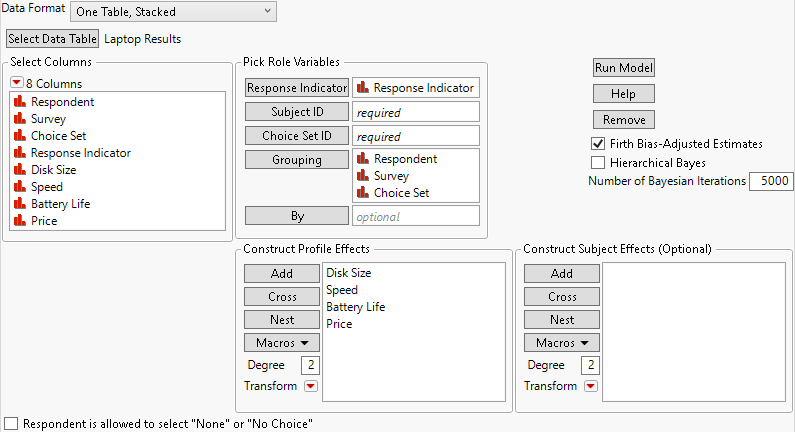There are three grouping variables, Respondent, Survey, and Choice Set, because there are multiple surveys and respondents.

3. Click Run Model.

Figure 18.11 Initial Analysis of the Final Laptop Design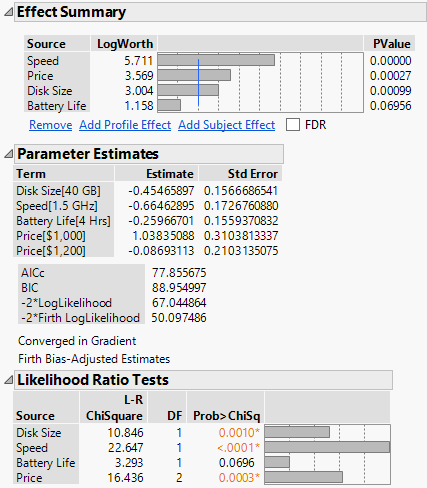The Effect Summary and Likelihood Ratio Tests outlines indicate that Disk Size, Speed, and Price are significant at the 0.05 level, and that Battery Life is marginally significant.

### Find Unit Cost and Trade Off Costs

Next, use the Profiler to see the utility value and how it changes as the laptop attributes change.

1. Click the Choice Model red triangle and select Utility Profiler.

Figure 18.12 Utility Profiler at Price = \$1000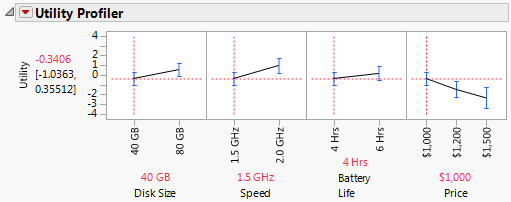When each attribute value is set to its lowest value, the Utility value is –0.3406. The first thing that you want to do is determine the unit utility cost.

2. Move the slider for Price to \$1,500.

Figure 18.13 Utility Profiler at Price = \$1500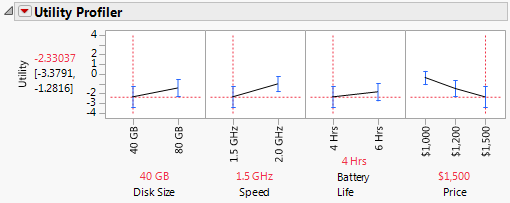When Price changes from \$1,000 to \$1,500, the Utility changes from –0.3406 to –2.3303. That is, raising the price of a laptop by \$500.00 lowers the utility (or desirability) approximately 2 units. Therefore, you can estimate the unit utility cost to be approximately \$250.00.

With this unit utility cost estimate, you can now vary the other attributes, note the change in utility, and find an approximate monetary value associated with attribute changes. For example, the most significant attribute is Speed (Figure 18.11).

3. In the Utility Profiler, set Price back to \$1,000, its lowest value, and change Speed to 2.0 GHz, its higher value.

Figure 18.14 Utility Value of Higher Speed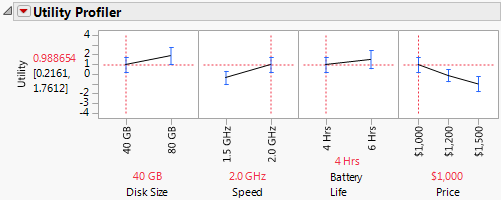The Utility value changes from the original value shown in Figure 18.12 of –0.3406 to 0.9886, for a total change of 1.3292 units. Using the utility cost estimate or \$250.00, the increase in price for a 2.0 GHz laptop over a 1.5 GHz laptop can be computed to be 1.3292*\$250.00 = \$332.30. This is the dollar value that the Choice study indicates that the manufacturer can use as a basis for pricing this laptop attribute. You can make similar calculations for the other attributes.

Want more information? Have questions? Get answers in the JMP User Community (community.jmp.com).